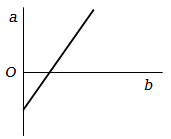# NEET Physics Kinetic Theory of Gases Questions Solved

The expansion of until mass of a perfect gas at constant pressure is shown in the diagram. Here1. a = volume, b = $°\mathrm{C}$ temperature

2. a = volume, b = $\mathrm{K}$ temperature

3. a = $°\mathrm{C}$ temperature, b = volume

4. a = $\mathrm{K}$ temperature, b = volume